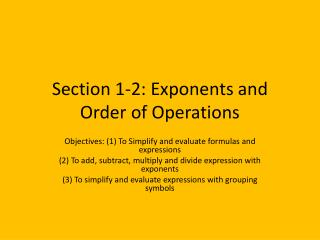Download PresentationSection 1-2: Exponents and Order of Operations

# Section 1-2: Exponents and Order of Operations

Download Presentation## Section 1-2: Exponents and Order of Operations

- - - - - - - - - - - - - - - - - - - - - - - - - - - E N D - - - - - - - - - - - - - - - - - - - - - - - - - - -
##### Presentation Transcript

1. Section 1-2: Exponents and Order of Operations Objectives: (1) To Simplify and evaluate formulas and expressions (2) To add, subtract, multiply and divide expression with exponents (3) To simplify and evaluate expressions with grouping symbols

2. Definitions • To simplify a numerical expression, you replace it with its simplest name. • An exponent tells how many times a number, the base, is used as a factor. • A power has two parts, a base and an exponent • You evaluate an algebraic expression by substituting a given number for each variable. Then Simplify the numerical expression using the order of operations.

3. Order of Operations Please, excuse my dear Aunt Sally. • Please • Parenthesis ( ), or other grouping symbols like braces { } and brackets [ ] • Excuse • Exponents • My • Multiplication • Dear • Division • Aunt • Addition • Sally • Subtraction

4. Order of Operations • This is the order in which you must begin evaluating expressions. • Multiplication and division are inverse operations (they undo each other) and therefore are done by the order in which they appear in the expression • Addition and subtraction are inverse operations and are done by the order in which they appear in the expression

5. Simplifying a Numerical Expression Simplify 25 – 8 • 2 + 32 25 – 8 • 2 + 9 25 – 16 + 9 9 + 9 18 • Which operation appears 1st? • Exponents! Find 32 • Now, multiplication • Subtraction comes first because add/subt is done by whichever comes first in the expression • And Add • 18 is the simplified answer

6. Evaluating an Algebraic Expression Evaluate 3a – 23 ÷ b a = 7 b = 4 3(7) – 23 ÷ (4) 3(7) – 8 ÷ 4 21 – 2 19 • First replace all variables with their numerical counterparts with parenthesis • Exponents first • Multiplication AND Division • Subtraction • All Done

7. Real World Examples (Word Problems) Your favorite pair of sneakers are on sale for \$59.00. There is a 6% sales tax in your state with any purchase. Find the total cost of the sneakers. Use the following formula: C = p + r• p C is the cost P is the price R is the sales tax rate

8. Real World Examples (Word Problems) C = 59 + (0.06)(59) C = 59 + 3.54 C = 62.54 You’re done! • Replace with actually values. • Order of operations tells us multiply first. • Now add • Easy, right?

9. Simplifying an Expression with Parenthesis Simplify 15(13 – 7) ÷ (8 – 5) 15(6) ÷ (3) 90 ÷ 3 30 All done! • Simplify the stuff inside the parenthesis first. • Remember that no symbol between a number outside a parenthesis and the parenthesis means multiply • Now divide

10. Evaluating Expressions with Exponents Evaluate each expression for c = 15 and d = 12 • (cd)2 [(15)(12)]2 (180)2 32,400 • Substitute 15 for c and 12 for d • Simplify the parenthesis first • Now raise it to the exponent

11. Evaluating Expressions with Exponents Evaluate each expression for c = 15 and d = 12 • cd2 (15)(12)2 (15)(144) 2,160 • Substitute 15 for c and 12 for d • Raise 12 to the 2nd power first • Now multiply

12. Simplifying an Expression Simplify 2[(13 – 7)2 ÷ 3] 2[(6)2 ÷ 3] 2[36 ÷ 3] 2 [ 12 ] 24 And you are done! • 1st simplify the parenthesis • 2nd Simplify the power • Simplify the brackets • Multiply

13. Real World Problem Solving:Urban Planning A neighborhood association turned a vacant lot into a park. The park is shaped like the trapezoid to the right. Use the formula to find the area of the lot. b1 = 100 ft h = 130 ft b2 = 200 ft

14. Real World Problem Solving:Urban Planning We know: The area of the park is 19,500 ft2 • Substitute 130 for h, 100 for b1 and 200 for b2 • Simplify the numerator • Simplify the fraction • Multiply